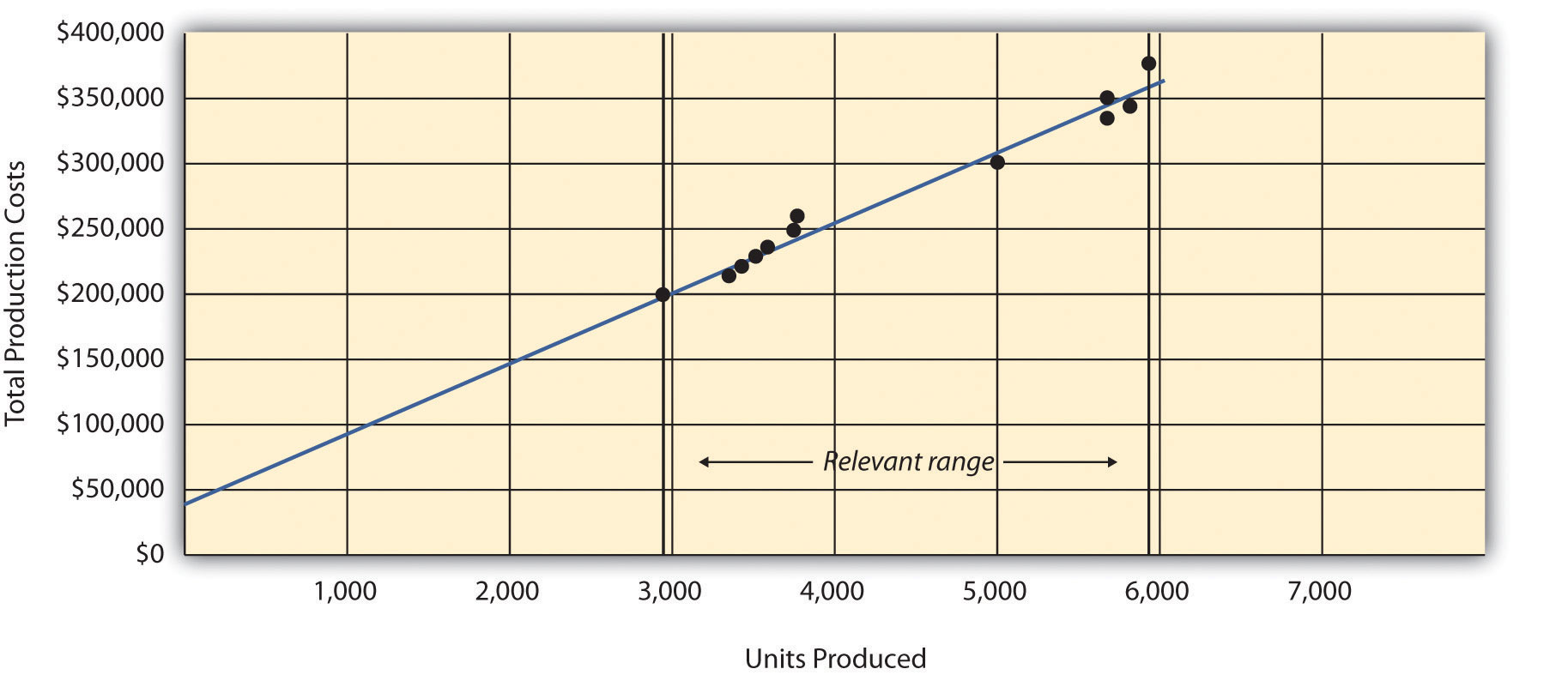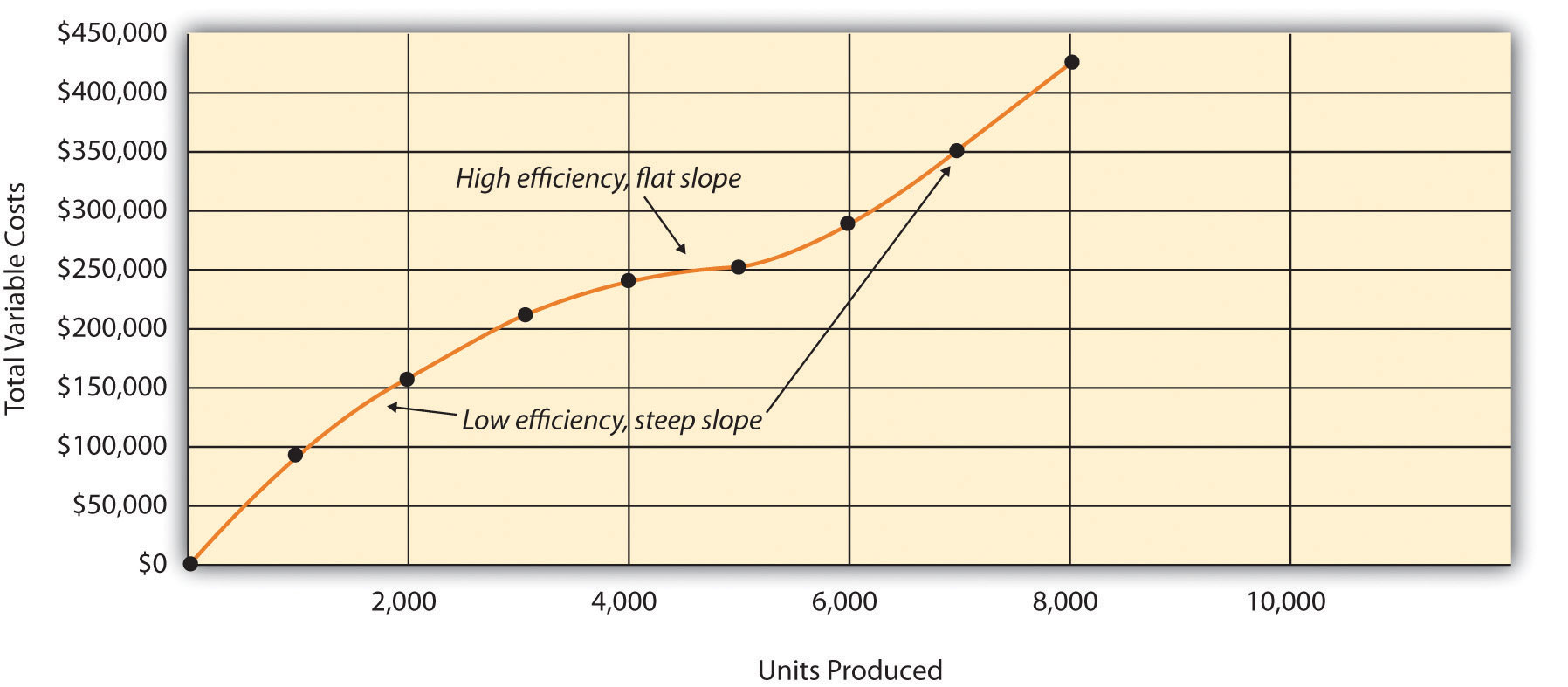## 5.4 The Relevant Range and Nonlinear Costs

### Learning Objective

1. Understand the assumptions used to estimate costs.

Question: Bikes Unlimited is making an important assumption in estimating fixed and variable costs. What is this important assumption and why might it be misleading?

Answer: The assumption is that total fixed costs and per unit variable costs will always be at the levels shown in Table 5.5 "Cost Equations for Bikes Unlimited" regardless of the level of production. This will not necessarily hold true under all circumstances.

For example, let’s say Bikes Unlimited picks up a large contract with a customer that requires producing an additional 30,000 units per month. Do you think the cost equations in Table 5.5 "Cost Equations for Bikes Unlimited" would lead to accurate cost estimates? Probably not, because additional fixed costs would be incurred for facilities, salaried personnel, and other areas. Variable cost per unit would likely change also since additional direct labor would be required (either through overtime, which requires overtime pay, or by hiring more employees who are less efficient as they learn the process), and the volume of parts purchased from suppliers would increase, perhaps leading to reductions in per unit costs due to volume discounts for the parts.

As defined earlier, the relevant range is a term used to describe the range of activity (units of production in this example) for which cost behavior patterns are likely to be accurate. Because the historical data used to create these equations for Bikes Unlimited ranges from a low of 2,900 units in January to a high of 5,900 units in April (see Table 5.4 "Monthly Production Costs for Bikes Unlimited"), management would investigate costs further when production levels fall outside of this range. The relevant range for total production costs at Bikes Unlimited is shown in Figure 5.8 "Relevant Range for Total Production Costs at Bikes Unlimited". It is up to the cost accountant to determine the relevant range and make clear to management that estimates being made for activity outside of the relevant range must be analyzed carefully for accuracy.

Figure 5.8 Relevant Range for Total Production Costs at Bikes UnlimitedRecall that Bikes Unlimited estimated costs based on projected sales of 6,000 units for the month of August. Although this is slightly higher than the highest sales of 5,900 units in April, Susan (cost accountant) determined that Bikes Unlimited had the production capacity to produce 6,000 units without significantly affecting total fixed costs or per unit variable costs. Thus she determined that a sales level of 6,000 units was still within the relevant range. However, Susan also made Eric (CFO) aware that Bikes Unlimited was quickly approaching full capacity. If sales were expected to increase in the future, the company would have to increase capacity, and cost estimates would have to be revised.

Question: Another important assumption being made by Bikes Unlimited is that all costs behave in a linear manner. Variable, fixed, and mixed costs are all described and shown as a straight line. However, many costs are not linear and often take on a nonlinear pattern. Why do some costs behave in a nonlinear way?

Answer: Assume the pattern shown in Figure 5.9 "Nonlinear Variable Costs" is for total variable production costs. Consider this: Have you ever worked a job where you were very slow at first but improved rapidly with experience? If a company produces just a few units each month, workers (direct labor) do not gain the experience needed to work efficiently and may waste time and materials. This has the effect of driving up the per unit variable cost. Recall that the slope of the line represents the unit cost; thus, when the unit cost increases, so does the slope. If the company produces more units each month, workers gain experience resulting in improved efficiency, and the per unit cost decreases (both in materials and labor). This causes the total cost line to flatten out a bit as the slope decreases. This is fine until the company starts to reach its limit in how much it can produce (called capacity). Now the company must hire additional inexperienced employees or pay its current employees overtime, which once again drives up the cost per unit. Thus the slope begins to increase.

Figure 5.9 Nonlinear Variable CostsAlthough this is probably a more accurate description of how variable costs actually behave for most companies, it is much simpler to describe and estimate costs if you assume they are linear. As long as the relevant range is clearly identified, most companies can reasonably use the linearity assumption to estimate costs.

### Key Takeaway

• Two important assumptions must be considered when estimating costs using the methods described in this chapter.

1. When costs are estimated for a specific level of activity, the assumption is that the activity level is within the relevant range.
2. Costs are estimated assuming that they are linear.

Both assumptions are reasonable as long as the relevant range is clearly identified, and the linearity assumption does not significantly distort the resulting cost estimate.

### Review Problem 5.8

1. Using the data in Note 5.21 "Review Problem 5.5", identify the relevant range.
2. Why is it important to determine the relevant range?

Solution to Review Problem 5.8

1. The relevant range, the range of activity for which cost estimates are more likely to be accurate, is from 150 units (lowest activity level) to 450 units of production (highest activity level).
2. Identifying the relevant range when estimating costs is important because if a cost estimate is being made for activity outside of the relevant range, total fixed costs and per unit variable costs may be different from those described in the cost equation. For example, if production is doubled, additional factory space may be needed, resulting in higher fixed costs.